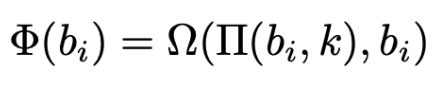# RITSEC CTF 2019 - CictroKDF

## Description

The flag is encrypted and then base64’d. Here it is: E2zVOQfaRwYoMiTapzA03RQJJmtq1KuHTCuWGdVo/+w=

To decrypt it you will need to implement the amazing Key Derivation Function called CictroKDF (not to be confused with the well-known CictroHash). After a key has been derived you can use it with AES ECB mode to decrypt the flag.

The message for the KDF is: Are you silly? I’m still gunna send it!!

Wrap the flag in RITSEC{}

Author: cictrone

## Hints

Hint: example setup Key: “Totally not a black box, I swear” Plaintext: “not a black box!” Ciphertext (base64’ed): GywB/y8LEXN335lUf6QWzA==

Hint2: pi_func(ord(‘a’), 0) = 42 pi_func(pi_func(ord(‘a’), 0), 1) = 70 pi_func(ord(‘b’), 0) = 46 pi_func(pi_func(ord(‘b’), 0), 1) = 228 pi_func(ord(‘d’), 0) = 136 pi_func(pi_func(ord(‘d’), 0), 1) = 92 pi_func(ord(‘d’), 50) = 168 pi_func(pi_func(ord(‘d’), 50), 25) = 94

## Solution

So basically you just had to implement the Key Derivation Function which was specified in the PDF Document.

Once that is done, you simply derive a key from the secret text and decrypt the provided base64 data with AES128 in ECB Mode.

Though the actual challenge seemed to be how to figure out what was actually meant by the pseudo mathematical definitions. Because they were confusing and inaccurate we didn’t manage to solve this in time.

Two key errors we made during this were:

1. in pi_func every bit should be changed based on the original byte value, NOT the already permuted bits.
2. similar thing in the phi function, the first bi value is supposed to change every round and the second bi is always supposed to be the original value.Another fun fact, because (i mod 4)th slot in the state array simply gets replaced every round means that only the last 4 bytes of the initial message are significant for the derived key.

The secret text Are you silly? I'm still gunna send it!! works as well as it!!.

The full implementation can be found in cictroKDF.py

Flag: RITSEC{i am a person who dos the crypto}

## Exploit Code

from Crypto.Cipher import AES
import base64

def check(word):
return len(word) % 4 == 0

def calc(msg):
state = ['h','a','s','h']
for i in range(len(msg)):
state[i%4] = round(ord(msg[i]),i)
return expand(state)

def round(b,i):
a = b
for k in range(100):
a = om(pi(a,k),b)
return a

def phi(b,k):
return om(pi(b,k),b)

def om(x,y):
return x ^ y ^ 56

def pi(n, k):
bi = list(map(int, list(bin(n)[2:].zfill(8))))
nbi = [0,0,0,0,0,0,0,0]
bi[7-(k%8)] = 1
nbi = bi
nbi = bi ^ bi
nbi = bi ^ bi
nbi = bi
nbi = bi
nbi = bi
nbi = bi
nbi = bi ^ bi

return int(''.join([str(x) for x in nbi]),2)

def exp(w,l):
return pi(w^w^w[l-1]^w[l],101)

def expand(state):
out = state
while len(out) < 19:
out.append(exp(out,len(out)-1))
return out[3:]

message = "Are you silly? I'm still gunna send it!!"
#message = "it!!"
assert check(message)
out = calc(message)
key =''.join([chr(x) for x in out])
cipher = AES.new(key,AES.MODE_ECB)
ct = base64.b64decode('E2zVOQfaRwYoMiTapzA03RQJJmtq1KuHTCuWGdVo/+w=')
pt = cipher.decrypt(ct)

print "key: "+key
print "plaintext: "+pt
print "Flag: RITSEC{"+pt+"}"